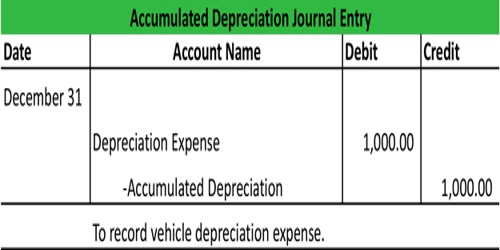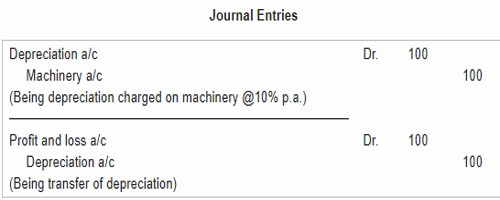Accounting

# Recording DepreciationRecording Depreciation

Depreciation is directly charged against the asset by debiting Depreciation account and crediting the Asset account. Depreciation account is closed by transferring to Profit and Loss account at the end of the year. The entries will be as under:

1) For the amount of depreciation to be provided at the end of the year:

Depreciation A/c….. To Asset A/c.

Dr… with the amount of depreciation.

2) For transferring the amount of depreciation at the end of the year.

Profit and Loss A/c….. To Depreciation A/c.

Dr…. with the amount of depreciation transferred

Asset Account will be shown at cost less depreciation i.e., written down value at the end of the year in the Balance sheet. Under this method, depreciation account is debited and asset account is credited with the amount of annual depreciation.

Example: Suppose, the cost of a machine is \$1,000 and its depreciation is 10% p.a. The entries to record the depreciation will be:This method of recording depreciation has now fallen into neglect. It has the consequence of reducing the balance shown in the ledger for the fixed asset so that over time, it might be very much fewer than the original cost. This makes it hard to recognize the original cost of fixed assets and means that, the only information that can be given is the value to which each fixed asset has been written down.